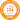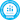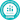# How to do Long Division: A Simple Step-By-Step Guide with Pictures

All PostsYou’ve guided your class through most of the big units: addition, division, subtraction, multiplication. But here’s another tricky one:

How to do long division.

A 2012 study published in Psychological Science found that 5th graders’ understanding of fractions and division could be directly linked with how well they understood algebra in high school and performed in higher-level math classes — even after controlling for various socio-economic factors.No pressure, right? If the thought of teaching long division gives you cold sweats and clammy palms, not to worry — we’ve done all the work for you.

## How to do long division in six steps

### 1. Review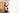The first step you should take is a step back.

For a 4th grade student, long division is a complicated mix of different operations. In order to successfully learn how to do long division, they need to review these fundamental concepts.

According to a French study, “the representation and retrieval of mathematical facts from long-term memory” is one of the most important factors in determining a student’s future mathematical success. According to the same study, long division is “a synthesis of all arithmetic knowledge.”

Make sure your students understand that multiplication is the product of repeated addition, and division is simply the opposite — repeated subtraction.

Use base 10 blocks or money to reinforce place value and number sense. Plan activities that ask students to create “fact families” in order to make sure students understand how different functions interact.

Use multiplication games and other math games to get students excited about learning and to build math confidence before you keep going.

### 2. Start simple

Let’s begin with a vocabulary lesson.There are lots of different parts in a long division equation. Make sure your students know what they mean and how to identify them.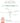The dividend is the number on the right side of the equation, under the line. It represents the amount being divided.

The divisor is the number on the left -- it’s the one doing the dividing.

The quotient is the number on the top. It represents the answer, or the number of units in each place value once the equation has been completed.

The remainder is the number on the top right. It represents the units left over that can’t be evenly divided into the quotient.First, introduce an equation that doesn’t have any remainders so students can get used to the format and start understanding the new vocabulary they’ve just learned: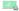Ask students how many times 2 fits into 4. This might be a tricky concept for them, so use the idea of sharing: If you want to share 4 objects between 2 people, how many objects does each person get?

Once they come up with the correct answer, put 2 above 4. Then, repeat the step with the second digit in the dividend.

Use these simple equations to reinforce place value. Teach students that when they’re asking how many times 2 can go into 4, they’re really asking how many times 2 goes into 40.

### 3. Remainder in the ones

Have your students practise the step above until they’re comfortable with the basic format. Then, it’s time to move on.

Instead of jumping right into an equation with remainders, start with another object lesson. Divide students into groups of three, four or six and give each group 50 cotton balls (or jelly beans, or pompoms, or marshmallows — whatever small object is accessible in your classroom).

Ask students to divide the objects up so each member of the group has an equal number, then watch and wait.

Eventually, they’ll understand they can’t divide it evenly and there will always be some objects left over. That’s where you come in to save the day and explain how to do long division with remainders.

First, show students a problem that has a remainder in the ones: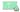Now, begin with the tens column and work through the problem: 5 goes into 5 exactly once, so there’s nothing left there. But how many times does 5 go into 7, and what do you do with the leftovers?

Show students the new steps:

• Divide the ones column dividend by the divisor
• Multiply the divisor by the quotient in the right place column
• Subtract the product from the ones column

The number they’re left with is the remainder. Make sure to model several problems as a class so students can begin to understand the steps and how to properly write their answers.

This is a good time in the lesson to teach students how to check their answers. Have them multiply the divisor by the quotient and add the remainder — the answer should be the same as the dividend they started with.

### 4. Remainder in the tens

Now it’s time for students to tackle problems where the divisor doesn’t fit neatly into the tensorones column. The steps are more or less the same, except for one new addition:

• Divide the tens column dividend by the divisor
• Multiply the divisor by the quotient in the tens place column
• Subtract the product from the divisor
• Bring down the dividend in the ones column and repeat.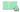Start with one-digit divisors and two-digit dividends to keep things simple. Remember that this is a totally new concept for students, so take the time to model problems on the board. Have a discussion about why the steps work, and help them understand how place value plays an important role in the process.

### 5. Introduce bigger numbers, gradually

So that’s it. Or is it?

Let students get comfortable with the formula and work on smaller problems. As they develop confidence and begin to understand how to do long division, start presenting them with problems that have a three-digit dividend, and then problems that have a two-digit divisor.

Remind students that the steps stay exactly the same no matter how big the problem is, and encourage them to use scrap paper to “guess-and-check” their multiplication as they go. This is a good spot to make sure that they’re not struggling and that they completely understand the relationship that division has with place value and multiplication.

For a refresher, check out this video from Khan Academy:

### 6. How to do long division with decimals

If you’ve covered all your content in the first five steps, congrats! Have students keep practicing how to do long division with large and small numbers, and keep reinforcing the relationship between division and other math concepts that they’re learning.But the process isn’t quite done yet — students need to understand how to do long division with decimals.To begin, go back to one of the fundamental concepts of division: place value. However, this time you’ll be going backwards instead of forwards.

Have students solve a problem as they normally would. When they get to the step where they would normally stop with a remainder, have them place a decimal point at the end of the quotient and the divided, and write in several zeros after the dividend.

Have them continue regular division steps for one or two places, bringing down the zeros.

Connect the decimal to fractions. Ask them to convert the quotient, with the decimal, to an improper fraction. This should help them understand the relationship between fractions and place value, and can be a good opportunity to go over fraction basics.

## How to do long division (without doing long division)

Congratulations! Your unit is coming to an end, and you’ve successfully taught your students how to do long division.

But did you know that there’s more than one way to divide large numbers? Teaching students other ways of checking their work is an important part of Common Core math standards and can improve student understanding of what long division actually means in a given context.

### Area models

Area models are a great way for visual learners to understand and conceptualize division while also enhancing number sense.

This method uses a grid to present the process of division as an area problem: for example, 148÷4 would be divided into a grid that is 4 units high, 148 square units in area, and an unknown number of units wide.

Students break up the grid into more manageable areas: 100 square units, 40 square units and 8 square units. 100÷4 is 25, 40÷4 is 10, and 8÷4 is 2. Those numbers go on the top of the area model and can be added up to reach the answer.

### Partial quotients

Similar to the area model, partial quotients encourage students to break division questions down into “friendlier” chunks. It helps students understand that division is finding how many times one number can go into another number.

Set up the problem (in this case, 450÷23) like a long division equation. Have student students multiply the divisor by 2 and 5 to use as an easy reference.

Ask how many times 23 goes into 400, but don’t look for the exact closest number: make it an easy number to work with, like 230 (ten times). Subtract 230 from 450 and put the 10 on the right to keep track of it.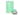Take the difference and subtract it from the dividend. The answer should be 220.

Ask how many times 23 goes into 220. 5 x 23 is 115, so subtract that from 220 and record the 5.

Keep going, multiplying and subtracting until the final number is too small. When you’ve reached that step, you’ve found your remainder! Add up the numbers in the right-hand column to find your quotient.

Partial quotients have a flexibility that long division doesn’t. Long division needs to be done precisely, but with partial quotients it’s possible to simply subtract the divisor from the dividend repeatedly and still come to the right answer.

Use this method to reinforce place value and the concept of division as repeated subtraction.

## Long division activities

The best way for students to learn how to do long division is to practice, practice, practice.

Here’s a list of eight activities that will get your class excited about long division and help them develop solid math skills.

### 1. Prodigy

Prodigy is a fun and engaging resource for in-class or at-home long division practice. Students explore a world filled with adventure, where success depends on correctly answering math questions.

Through the teacher dashboard, you can deliver lesson-aligned content based on the grade, skill or student. Then, students answer these questions in-game and provide you with real-time feedback on their learning and comprehension.

Encourage your students to practice all the math skills they’ve been learning in the classroom — including long division. Here's how you can use Prodigy to: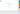Students play the engaging game-based platform, where they can collect pets, go on quests and battle with friends. And while they’re having fun, you’re helping them develop long division skills. It’s a win for everyone!

### 2. Life-sized long division

Bring math to life with a hands-on long division puzzle. Cut out squares of colorful paper with all the numbers that students need to solve a long division problem from start to finish. Use masking tape to make division lines on the floor, and give students the numbered cards.

Starting with a given equation, have students lay out all the cards in the correct order to solve the equation. This activity challenges students to slow down and think about their steps, and it’s especially helpful for a class that’s still looking to master the multiplication steps.

### 3. Long division bingo

Bingo is a classic for a reason. Each of the numbers on a student’s sheet should match up to a question that you have at the front of the classroom. Write the problem on the board, and then give students scratch paper and a chance to solve it and see if they have it on their card. As always, the first student to fill up a whole row, wins!

Challenge your students, but make sure you take enough time with this activity — some students might have trouble solving problems quickly, and can get frustrated or make mistakes if they’re not able to keep up.

### 4. Math books

Boost literacy and math learning at the same time with fun books covering tricky math concepts. Use them to explain division and remainders to students in a fun and engaging way, and even to cover more basic concepts before they start learning how to do long division.

Some math books that cover division include:

• A Remainder of One by Elinor J. Pinczes
• Bean Thirteen by Matthew McElligott
• The Doorbell Rang by Pat Hutchins

### 5. Get creative

Long division has lots of steps, and they have to be done in the right order to get to the correct answer. Students can get confused or frustrated when they don’t remember the steps, which negatively affects their math confidence and performance.

Challenge students to come up with their own unique way to remember how to do long division —divide, multiply, subtract and bring down— to get the creativity flowing in your classroom.

Have them create a poster, song, mnemonic device or even a small skit that they can present to their classmates. If they’re invested in finding a way to remember the steps, they’re more likely to learn quickly.

### 6. Long division relays

Turn long division practice into a fun classroom game with long division relays. Divide your class up into teams and make cards with long division problems on them.

Line up students in groups. Each group gets a card to start, and the first students complete the first set of steps for the problem they have.

When they’re done, the second student looks for errors and continues the problem. If they finish the problem, they can call for you to come check their work and trade the correct answer for a card with a new problem.

Keep going until every group has answered all their cards, and see which team wins!

7. Treasure chest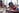This activity is a fun way for your class to celebrate finishing their unit on division. Get a few boxes and fill them with a small treat everyone in the class can enjoy. Include a list of multiplication problems that students must solve in groups to “unlock” the box.

For an added challenge, make it a code: Have every quotient match up to a letter of the alphabet so that students have to correctly decode a key phrase to open the box.

### 8. Worksheet generator

Worksheets are a tried-and-true staple of math class. Lucky for you, there are lots of websites that will do the work for you and generate a custom worksheet that will give your students the change to practice how to do long division. Here are a few of our favourites:

## Final thoughts on teaching students how to do long division

The most important thing to remember when teaching students how to do long division is to not rush through the material. It’s a big concept that’s different from anything they’ve learned before, and some (if not all) of your students might struggle at first.If you need to, go back to simpler equations and some of the earlier steps that we’ve outlined for you and work through them until your students feel confident. Keep on encouraging and challenging your students, and they’ll be ready to divide and conquer in no time!

Create or log in to your free teacher account on Prodigy – a game-based learning platform for math that’s easy to use for educators and students alike. Aligned with curricula across the English-speaking world, it’s used by millions of teachers and students.